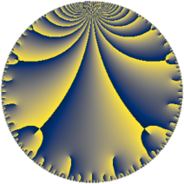# Properties

 Label 162.7.dLevel $162$ Weight $7$ Character orbit 162.d Rep. character $\chi_{162}(53,\cdot)$ Character field $\Q(\zeta_{6})$ Dimension $48$ Newform subspaces $7$ Sturm bound $189$ Trace bound $7$

# Related objects

## Defining parameters

 Level: $$N$$ $$=$$ $$162 = 2 \cdot 3^{4}$$ Weight: $$k$$ $$=$$ $$7$$ Character orbit: $$[\chi]$$ $$=$$ 162.d (of order $$6$$ and degree $$2$$) Character conductor: $$\operatorname{cond}(\chi)$$ $$=$$ $$9$$ Character field: $$\Q(\zeta_{6})$$ Newform subspaces: $$7$$ Sturm bound: $$189$$ Trace bound: $$7$$ Distinguishing $$T_p$$: $$5$$

## Dimensions

The following table gives the dimensions of various subspaces of $$M_{7}(162, [\chi])$$.

Total New Old
Modular forms 348 48 300
Cusp forms 300 48 252
Eisenstein series 48 0 48

## Trace form

 $$48 q + 768 q^{4} - 1200 q^{7} + O(q^{10})$$ $$48 q + 768 q^{4} - 1200 q^{7} - 8400 q^{13} - 24576 q^{16} - 14520 q^{19} + 7200 q^{22} + 96312 q^{25} - 76800 q^{28} - 70680 q^{31} + 54720 q^{34} - 59808 q^{37} - 208380 q^{43} + 270144 q^{46} - 539064 q^{49} + 268800 q^{52} - 1751976 q^{55} - 796320 q^{58} + 56112 q^{61} - 1572864 q^{64} - 528924 q^{67} - 185760 q^{70} + 241800 q^{73} - 232320 q^{76} + 3161292 q^{79} - 787104 q^{82} - 2051784 q^{85} - 230400 q^{88} - 4259328 q^{91} + 1473696 q^{94} - 3107676 q^{97} + O(q^{100})$$

## Decomposition of $$S_{7}^{\mathrm{new}}(162, [\chi])$$ into newform subspaces

Label Dim $A$ Field CM Traces $q$-expansion
$a_{2}$ $a_{3}$ $a_{5}$ $a_{7}$
162.7.d.a $4$ $37.269$ $$\Q(\sqrt{-2}, \sqrt{-3})$$ None $$0$$ $$0$$ $$0$$ $$-778$$ $$q+(2\beta _{1}-2\beta _{3})q^{2}+(2^{5}-2^{5}\beta _{2})q^{4}+\cdots$$
162.7.d.b $4$ $37.269$ $$\Q(\sqrt{-2}, \sqrt{-3})$$ None $$0$$ $$0$$ $$0$$ $$-4$$ $$q+(\beta _{1}-\beta _{3})q^{2}+(2^{5}-2^{5}\beta _{2})q^{4}+30\beta _{1}q^{5}+\cdots$$
162.7.d.c $4$ $37.269$ $$\Q(\sqrt{-2}, \sqrt{-3})$$ None $$0$$ $$0$$ $$0$$ $$410$$ $$q+(-\beta _{1}+\beta _{3})q^{2}+(2^{5}-2^{5}\beta _{2})q^{4}+\cdots$$
162.7.d.d $4$ $37.269$ $$\Q(\sqrt{-2}, \sqrt{-3})$$ None $$0$$ $$0$$ $$0$$ $$968$$ $$q+(-4\beta _{1}+4\beta _{3})q^{2}+(2^{5}-2^{5}\beta _{2})q^{4}+\cdots$$
162.7.d.e $8$ $37.269$ $$\Q(\zeta_{24})$$ None $$0$$ $$0$$ $$0$$ $$-836$$ $$q+\zeta_{24}^{5}q^{2}+2^{5}\zeta_{24}^{2}q^{4}+(5\zeta_{24}-5\zeta_{24}^{3}+\cdots)q^{5}+\cdots$$
162.7.d.f $8$ $37.269$ $$\Q(\zeta_{24})$$ None $$0$$ $$0$$ $$0$$ $$4$$ $$q+4\zeta_{24}^{3}q^{2}+(2^{5}-2^{5}\zeta_{24})q^{4}+(95\zeta_{24}^{5}+\cdots)q^{5}+\cdots$$
162.7.d.g $16$ $37.269$ $$\mathbb{Q}[x]/(x^{16} + \cdots)$$ None $$0$$ $$0$$ $$0$$ $$-964$$ $$q+4\beta _{8}q^{2}+(2^{5}-2^{5}\beta _{2})q^{4}+(-12\beta _{8}+\cdots)q^{5}+\cdots$$

## Decomposition of $$S_{7}^{\mathrm{old}}(162, [\chi])$$ into lower level spaces

$$S_{7}^{\mathrm{old}}(162, [\chi]) \cong$$ $$S_{7}^{\mathrm{new}}(9, [\chi])$$$$^{\oplus 6}$$$$\oplus$$$$S_{7}^{\mathrm{new}}(18, [\chi])$$$$^{\oplus 3}$$$$\oplus$$$$S_{7}^{\mathrm{new}}(27, [\chi])$$$$^{\oplus 4}$$$$\oplus$$$$S_{7}^{\mathrm{new}}(54, [\chi])$$$$^{\oplus 2}$$$$\oplus$$$$S_{7}^{\mathrm{new}}(81, [\chi])$$$$^{\oplus 2}$$